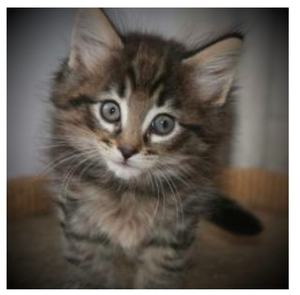## 混合模式

### 基本原理

$C_{mix} = f(C_{backdrop},\ C_{source})$

• $C_{mix}$：代表混合之后得到的颜色；
• $C_{backdrop}$：代表处于相对底层的颜色；
• $C_{source}$：代表处于相对上层的颜色；
• $f$：代表用于混合两种颜色的函数；

### 相关概念

• 颜色通道：由于数字图像存储像素颜色信息都是按不同颜色成分分别进行存储的，因此将图像中的某一成分单独拿出来组成的灰度图像就是其中的一个颜色通道；例如常见的RGBA图像就有R，G，B，A四个颜色通道；

A channel in this context is the grayscale image of the same size as a color image, made of just one of these primary colors.

• 互补色：在RGB颜色模型中，一种颜色的互补色就是用白色减去该颜色所得到的颜色；可以这样描述：

$C_{互补} = 1 - C$

• 可分离混合模式Separable blend modes）：这里的可分离指的是对不同的颜色通道进行混合然后再合并得到的混合效果是一样的，即不同的颜色通道只依赖于对应的颜色通道信息

### 混合函数

#### 可分离

• 正常（normal）：默认情况，即上层像素直接覆盖下层像素；

$f(C_b,\ C_s) = C_s$

• 正片叠底（multiply）：上层像素与下层像素各通道值进行相乘，得到的图像较原图像会偏暗；当一方像素为黑色时得到的像素自然也是黑色，反之若一方像素为白色得到的像素则为另一方像素原值；

$f(C_b,\ C_s) = C_b * C_s$

• 滤色（screen）：先将两者的互补进行正片叠底，然后再取其互补；这种情况通常会使图像变得更亮，当一方像素为黑色时得到的像素值就是另一方像素值，而当一方像素为白色时得到的像素也是白色；因此最后混合得到图像会有一个特点：颜色值再低也不会低于两者中的任何一个

\begin{aligned} f(C_b,\ C_s) &= 1 - [(1 - C_b) * (1 - C_s)]\\[1em] &= C_b + C_s - C_b * C_s \end{aligned}

• 变暗（darken）：取两者中各自通道中最小的值

$f(C_b,\ C_s) = min(C_b, C_s)$

• 变亮（lighten）：取两者中各自通道中最大的值

$f(C_b,\ C_s) = max(C_b, C_s)$

• 颜色减淡（color-dodge）：作用是提高底层颜色亮度，然后反射上层颜色，最后达到提高图像亮度的目的；

$f(C_b,\ C_s) = \begin{cases} 0, & C_b = 0 \\[1em] 1, & C_s = 1 \\[1em] min(1,\ \large\frac{C_b}{1 - C_s}), & else \end{cases}$

• 颜色加深（color-burn）：跟color-dodge作用相反，会降低底层颜色亮度，然后反射上层颜色，降低图像的亮度

$f(C_b,\ C_s) = \begin{cases} 1, & C_b = 1 \\[1em] 0, & C_s = 0 \\[1em] 1 - min(1,\ \large\frac{1 - C_b}{C_s}), & else \end{cases}$

• 强光（hard-light）：颜色变亮或变暗取决于上层的像素颜色，暗则更暗，亮则更亮；效果类似于在底层图像上加上高（镜面）反射光

$f(C_b,\ C_s) = \begin{cases} f_{multiply}(C_b,\ 2 * C_s), & C_s \le 0.5 \\[1em] f_{screen}(C_b,\ 2 * Cs - 1), & else \end{cases}$

• 弱光（soft-light）：颜色变亮或变暗取决于上层的像素颜色，效果类似于在底层图像上加上漫反射光

$D(C) = \begin{cases} ((16 * C - 12) * C + 4) * C, & C \le 0.25 \\[1em] \sqrt{C}, & else \end{cases}\\[2em] f(C_b,\ C_s) = \begin{cases} C_b - (1 - 2 * C_s) * C_b * (1 - C_b), & C_s \le 0.5 \\[1em] C_b + (2 * Cs - 1) * (D(C_b) - C_b), & else \end{cases}$

• 叠加（overlay）：将两者互换位置，然后再进行强光处理；因此类似于给上层图像加了镜面反射光；

$f(C_b,\ C_s) = f_{hard-light}(C_s,\ C_b)$

• 差值（difference）：获取两者各自通道之间的差值；

$f(C_b,\ C_s) = | C_b - C_s |$

• 排除（exclusion）：从结果来看，exclusion等于滤色效果减去正片叠底；从效果上看则与差值类似，但是具有更低的对比度；

$f(C_b,\ C_s) = C_b + C_s - 2 * C_b * C_s$

#### 不可分离

1. RGB转为HSL颜色模型；
2. 然后对H，S，L分量进行处理；
3. 最后再最转回RGB颜色模型；

• $Lum(C)$：获取颜色灰度值；
• $ClipColor(C)$：将颜色值范围进行校正，保证颜色分量在[0, 1]之间；
• $SetLum(C,\ l)$：将颜色值转为灰度为l的颜色；
• $Sat(C)$：获取RGB颜色的饱和度（即HSL中的S分量）；
• $SetSat(C,\ s)$：将RGB颜色中的饱和度转为s

• 色相（hue）：使用底层颜色的SL分量，加上上层颜色的H分量，得到一个新的颜色；

$f(C_b,\ C_s) = SetLum(SetSat(C_s,\ Sat(C_b)),\ Lum(C_b))$

• 饱和度（saturation）：使用底层颜色的HL分量，加上上层颜色的S分量，得到一个新的颜色；

$f(C_b,\ C_s) = SetLum(SetSat(C_b,\ Sat(C_s)),\ Lum(C_b))$

• 颜色（color）：使用上层颜色的HS分量，加上底层的颜色的L分量，得到一个新的颜色；

$f(C_b,\ C_s) = SetLum(C_s,\ Lum(C_b))$

• 明度（luminosity）：使用底层颜色的HS分量，加上上层的颜色的L分量，得到一个新的颜色；

$f(C_b,\ C_s) = SetLum(C_b,\ Lum(C_s))$

## CSS中混合模式相关的属性

css3中引入了两个与混合模式有关的属性：

• background-blend-mode：用于指定元素背景（包括背景图和背景色）之间的混合模式；
• mix-blend-mode：用于指定元素与其叠层之下的元素/层级之间的混合模式；

## 混合模式的应用

### 滤色模式叠加特效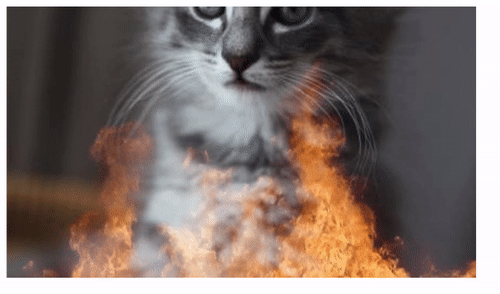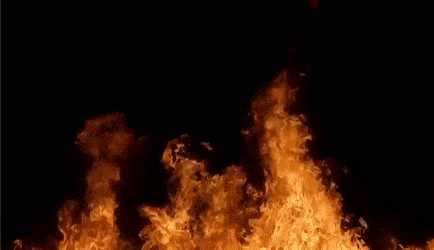### 滤色模式制作图片背景的文字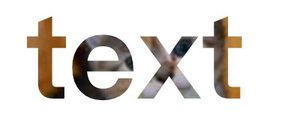• 文字单独设置成一层，然后设置mix-blend-mode: screen
• 文字颜色为黑色：因此可以对文字点阵区域保留底层的像素；
• 文字区域背景色为白色：因此文字点阵以外区域皆为白色；

### 渐变色文字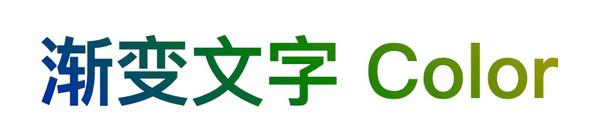### 自制滤镜

#### 正片叠底过滤颜色通道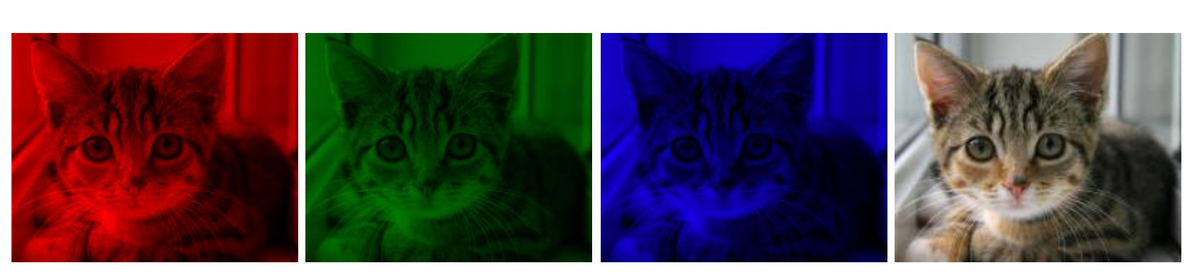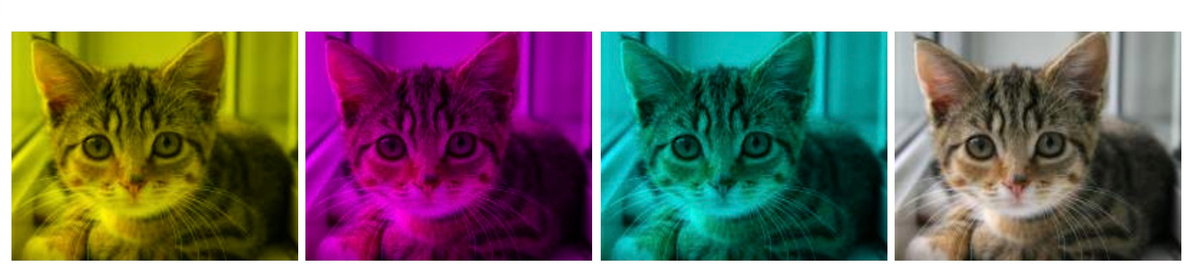### 晕影效果